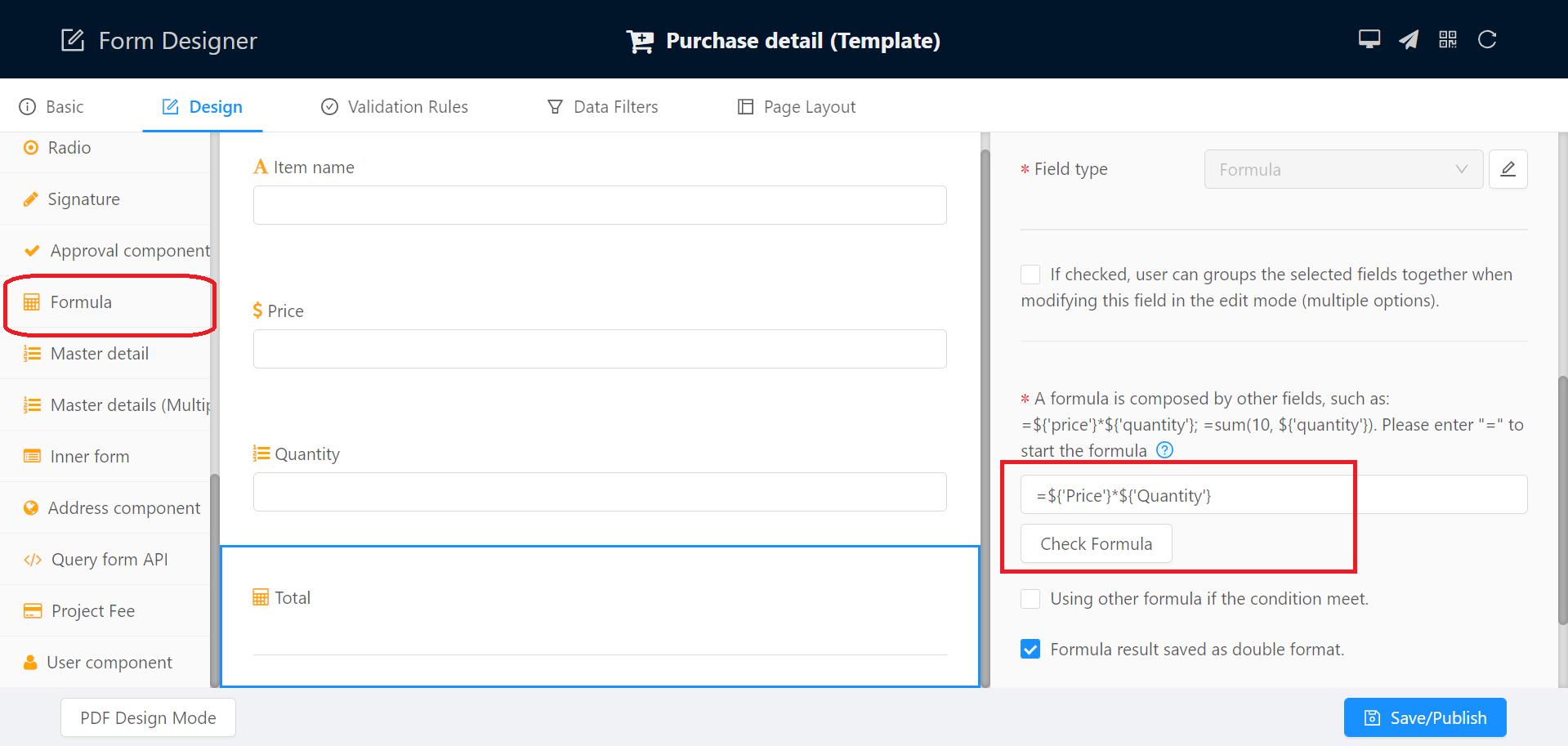Application form   ->   Formula

## Formula

CubeDrive allows the user to define the formula field type. With formulas, users can easily process some data calculation. It can be used to:

• Improve the efficiency to fill the form, automatically calculate the data, and display the data content based on some logic.
• Reduce error in the calculation process.

### Setup formula

When creating a new field, author can drag the Formula field type from the field list and drop to the center panel. You also need to set the formula properties on the right panel.To setup a formula, you need enter the format with "=" start in the formula text field.

Tip: user can setup another formula format based on certain pre-defined condition.

If the formula result is double (such as a currency result), please check the following setting. In APP, the double format formula will be able to process further calculate action.

• Formula result saved as double format.

#### Examples

• =\${'Number'}*\${'Number2'}*1.13
• =SUM(\${'Number'},\${'Number2'})
• =PRODUCT(\${'Number'},\${'Currency'})
• =INT(YEARFRAC(\${'birthdate'},TODAY()))
• =IF(\${'Number'}>12, "Teenager", "Kid")
• =CONCATENATE(\${'text1'},\${'text2'}, ...)
• =TEXT(minutes(\${'Date1'}, \${'Date2'}), 'h:mm')
• =INT(MINUTES(\${'Start'},\${'end'})/(60*24))+1
• =IF(AND(\${'total'}>0, ISBLANK(\${'manager'})), false, true)
• =IF(AND(\${'total'}>0, OR(ISBLANK(\${'manager'}), ISBLANK(\${'organization'}))), false, true)
• =IF(days(\${'Exp Contract End Date'}, '2022-01-01') > 0, true, false)
• =IF(AND(days(\${'EXPIRE-DT'}, today())>=0, days(\${'EXPIRE-DT'}, today())<=30), true, false)
```=concatenate('【',if(\${'role'}='employee','employee-',''),if(\${'role'}='manager','Manager-',''),'CubeDrive',if(\${'role'}='CEO','-CEO,',''),if(\${'role'}='employee','-employer,',''),if(\${'startDate'},TEXT(\${'startDate'},"MM/dd"),'Recently ') ,' run】', \${'type'},'，',\${'body'},'，',\${'rank'},'，',\${'total'},'，',\${'period'},'，',\${'location'},'，History：',\${'history'}, if(\${'extra'}, ''+\${'extra'}, ''), if(\${'interesting'}, ''+\${'interesting'}, ''), if(\${'code'}, ''+\${'code'}, ''))
```

### Functions

Here lists the supported formulas at this moment.

• + - * /
Syntax: =(\${'Number'}+\${'Number2'})*1.1
User + - * / to process the basic math calculation.
• ABS
Syntax: =ABS(\${'Number'})
Returns the absolute value of the given number.
• AND
Syntax: =AND(days(\${'EXPIRE-DT'}, today())>=0, days(\${'EXPIRE-DT'}, today())<=30)
Returns the calculated conditions result.
• AVERAGE
Syntax: =AVERAGE(\${'Number'},\${'Number2'}, ...)
Returns the average of the arguments. Number1, number2, ... are numerical values or ranges. Text is ignored.
• CONCATENATE
Syntax: =CONCATENATE(\${'text1'},\${'text2'}, ...)
Combines several text strings into one string.
• DAYS
Syntax: =DAYS(\${'endDate'}, \${'startDate'})
This function returns the number of days between those two dates. First argument is end date, second argument is start date. Example: =days(\${'Exp Contract End Date'}, '2022-01-01'); =DAYS(\${'endDate'}, today())
• DEGREES
Syntax: =DEGREES(\${'Number'})
This function converts radians into degrees.
• EXP
Syntax: =EXP(\${'Number'})
Returns a Double specifying e (the base of natural logarithms) raised to a power.
• FIXED
Syntax: =FIXED(\${'Number'}, 2)
Rounds a number to the specified number of decimals, formats the number in decimal format using a period and commas, and returns the result as text.
• IF
Syntax: =IF(\${'Number'}>12, "Teenager", "Kid")
Returns one value if a condition evaluates to TRUE and another value if it evaluates to FALSE. .
• INT
Syntax: =INT(\${'Number'})
Rounds a number down to the nearest integer.
• MAX
Syntax: =MAX(\${'Number'},\${'Number2'}, ...)
Returns the max of the arguments. Number1, number2, ... are numerical values or ranges.
• MIN
Syntax: =MIN(\${'Number'},\${'Number2'}, ...)
Returns the min of the arguments. Number1, number2, ... are numerical values or ranges.
• PRODUCT
Syntax: =PRODUCT(\${'Number'},\${'Number2'}, ...)
Returns the multiple of corresponding array numbers
• RAND
Syntax: =RAND()
Returns a random number between 0 and 1.
• SQRT
Syntax: =SQRT(\${'Number'})
Returns a positive square root.
• SQRTPI
Syntax: =SQRTPI(\${'Number'})
Returns a positive square root of (number * pi).
• SUM
Syntax: =SUM(\${'Number'},\${'Number2'}, ...)
Returns the sum of corresponding array numbers
• SUMPRODUCT
Syntax: =SUMPRODUCT(\${'Number'},\${'Number2'},...)
Product all numbers in a range of items.
• TONUMBER
Syntax: =TONUMBER(\${'Text'})
Extract numbers from the input TEXT.
• TODAY
Syntax: =TODAY()
Returns the current computer system date. TODAY is a function without arguments.
• YEARFRAC
Syntax: =YEARFRAC(\${'birthdate'},TODAY())
Calculates the fraction of the year represented by the number of whole days between two dates (the start_date and the end_date). Use the YEARFRAC worksheet function to identify the proportion of a whole year's benefits or obligations to assign to a specific term.